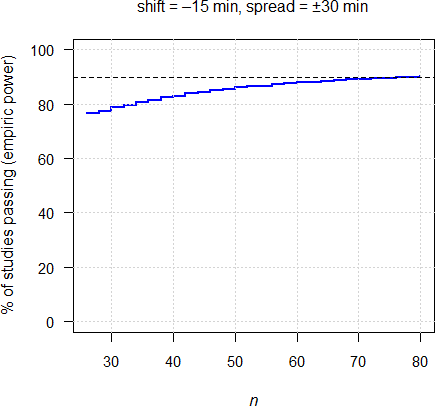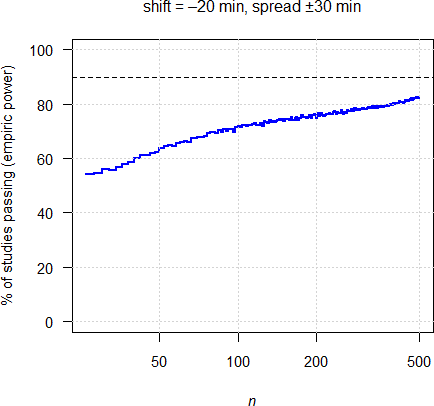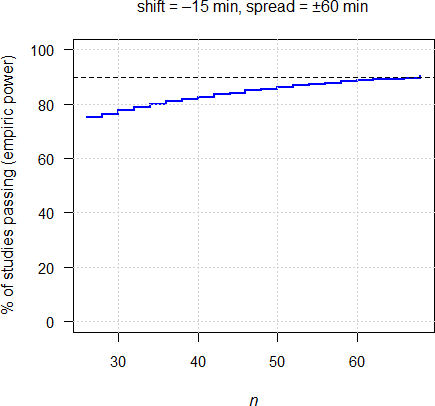## Preliminary simulations [BE/BA News]

Hi ElMaestro,

❝ 2. Do we use logs or not?

As the ‘Two Lászlós’ – who else? – wrote*

The concentrations rise more steeply before the peak than they decline following the true maximum response. Consequently, it is more likely that large observed concentrations occur after than before the true peak time ($$\small{T_\textrm{max}^\circ}$$).

Apart from this theoretical consideration they demonstrated in simulations that the distribution of the observed tmax is not only biased but skewed to the right.

I could confirm that in my studies. Given that, your idea of using logs in simulations (but not in the evaluation) is excellent! It turned out that a CV of 50% is not uncommon – even with a tight sampling schedule.

I set up simulations. Say, we have a drug with an expected median tmax of R of 1.5 hours. If we aim to power the study at 90% for a moderate CV of Cmax of 20% we need 26 subjects. Let’s sample every 15 minutes and assume a shift in tmax for T of 15 minutes (earlier). Result of 10,000 simulations:

Sample size based on conventional PK metric   BE limits    : 80.00% to 125.00%   theta0       : 95% (assumed T/R ratio)   CV           : 20% (assumed)   Power        : 90% (target)   n            : 26  (estimated)   Power        : 92% (achieved)   Sampling     : every 15 minutes   mu.tmax (R)  : 1.50 h    (assumed for R)   CV of tmax   : 50%       (assumed)   shift        : -15.0 min (assumed for T)   spread       : ±30.0 min Not equivalent if medians differ by more than 20% {-18 min, +18 min}   Empiric power: 76.00%

Oops!

How many subjects would we need to preserve our target power?

  n            : 78  (specified)   Power        : 100% Not equivalent if medians differ by more than 20% {-18 min, +18 min}   Empiric power: 90.71%

Three times as many subjects. Not funny.Another issue is the assumed shift in location, which is nasty. I tried –20 minutes (instead of –15).I stopped the iterations with 500 (‼) subjects (power ≈82%). I’m not sure whether 90% is attainable even with a more crazy sample size. Note that the x-axis is in log-scale.

On the other hand, the spread is less important, since we are assessing medians. In other words, extreme values are essentially ignored. Then with the original shift of –15 minutes but a spread of ±60 minutes (instead of 30), we have already ≈80% power with 36 subjects and ≈90% with 66.Is this really the intention? The wider the range in tmax, the more easily products will pass. Counterintuitive.

Furthermore, as a consequence of the skewed distribution power curves are not symmetrical around zero – what the PKWP possibly (or naïvely?) assumed. It reminds me on the old days when the BE-limits were 80–120% and maximum power was achieved at a T/R-ratio of ≈0.98 (see there).Consequently, a ‘faster’ test will more likely pass than a ‘slower’ one. That’s not my understanding of equivalence. Guess the Type I Error.

• Tóthfálusi L, Endrényi L. Estimation of Cmax and Tmax in Populations After Single and Multiple Drug Ad­mi­ni­stra­tion. J Pharma­co­kin Pharma­codyn. 2003; 30(5): 363–85. doi:10.1023/b:jopa.0000008159.97748.09.

Dif-tor heh smusma 🖖🏼 Довге життя Україна!Helmut SchützThe quality of responses received is directly proportional to the quality of the question asked. 🚮
Science QuotesIng. Helmut Schütz